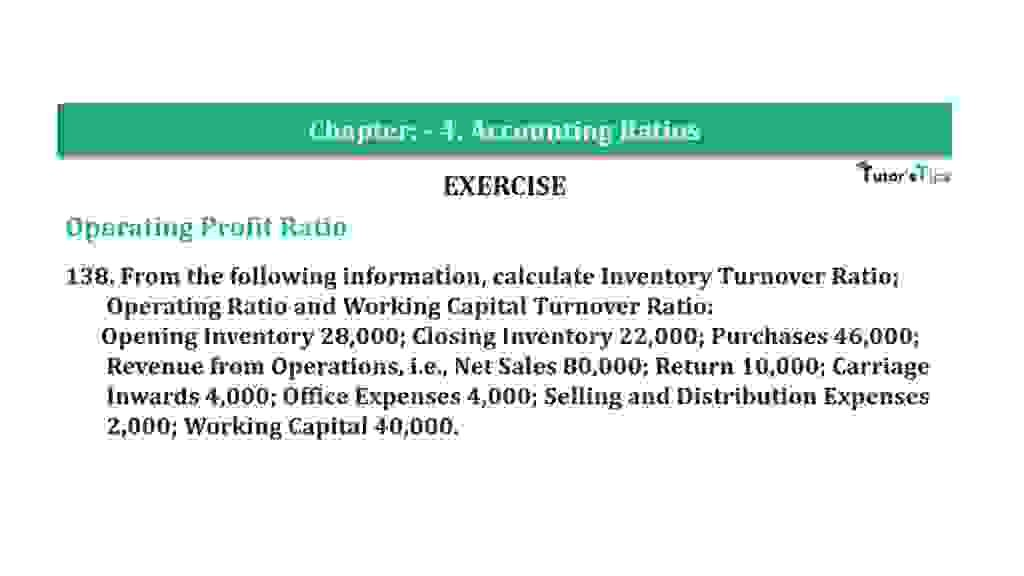# Question 138 Chapter 4 of +2-B – T.S. Grewal 12 ClassQuestion 138 Chapter 4 of +2-B

Miscellaneous

138. From the following information, calculate Inventory Turnover Ratio;
Operating Ratio and Working Capital Turnover Ratio:
Opening Inventory 28,000; Closing Inventory 22,000; Purchases 46,000;
Revenue from Operations, i.e., Net Sales 80,000; Return 10,000; Carriage
Inwards 4,000; Office Expenses 4,000; Selling and Distribution Expenses
2,000; Working Capital 40,000.

### The solution of Question 138 Chapter 4 of +2-B: –

 Cost of Goods Sold = Opening Inventory + Purchases + Carriage Inwards − Closing Inventory = Rs 28,000 + Rs 46,000 + Rs 4,000 – Rs 22,000 = Rs. 56,000

 Average Inventory = Opening Inventory + Closing Inventory 2
 Current Ratio = Rs. 28,000 + Rs 22,000 2 = Rs 25,000

 Inventory Turnover Ratio = Cost of Goods Sold Average Inventory
 = Rs. 56,000 Rs. 25,000 = 2.24 Times

 Operating Expenses = Office Expenses + Selling and Distribution Expenses = Rs 4,000 − Rs 2,000 = Rs 6,000 Operating Cost = Cost of Goods Sold + Operating Expenses = Rs 56,000 + Rs 62,000 = Rs 62,000
 Operating Ratio = Operating Cost X 100 Net Sales
 Operating Ratio = Rs 62,000 X 100 Rs 80,000 = 77.5%
 Working Capital = Rs 40,000 Net Sales = Rs 80,000
 Working Capital Turnover Ratio = Net Sales Working Capital
 = Rs 80,000 Rs 40,000 = 2 Times

Balance Sheet: Meaning, Format & Examples

Thanks, Please Like and share with your friends

Comment if you have any question.

Also, Check out the solved question of previous Chapters: –

### T.S. Grewal’s Double Entry Book Keeping (Vol. II: Accounting for Companies)T.S. Grewal’s Analysis of Financial Statements